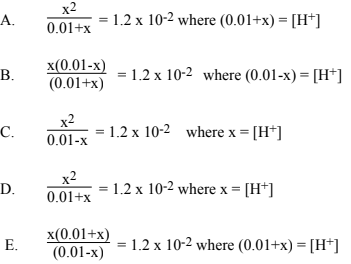# Problem: H2SO4 is a diprotic acid. For H2SO4: Ka1 is large and Ka2 = 1.2 x 10-2Which of the following equations will allow you to calculate [H+] in 0.010 M H2SO4?

###### FREE Expert Solution

We’re being asked to identify the equation that would calculate the [H+] in 0.010 M H2SO4.

H2SO4

can donate two protons (H+) → diprotic acid
two equilibrium equations → two equilibrium constants

Ka1 → large
Ka2 = 1.2x10-2

89% (303 ratings)###### Problem Details

H2SO4 is a diprotic acid. For H2SO4: Ka1 is large and Ka2 = 1.2 x 10-2

Which of the following equations will allow you to calculate [H+] in 0.010 M H2SO4?